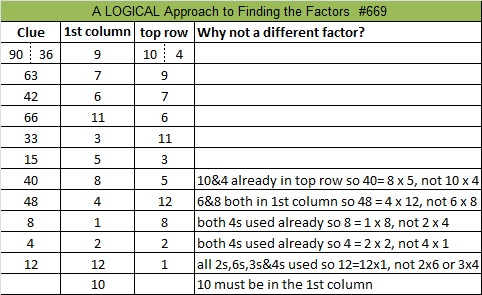# 669 and Level 4

Since all its digits are divisible by 3, obviously 669 is divisible by 3.

222 + 223 + 224 = 669. Thus 669 is the sum of 3 consecutive numbers. Prime factor 223 is the middle number in the sum.

Also consecutive numbers 109 + 110 + 111 + 112 + 113 + 114 = 669Print the puzzles or type the solution on this excel file: 12 Factors 2015-11-02

—————————————————————————————————

• 669 is a composite number.
• Prime factorization: 669 = 3 x 223
• The exponents in the prime factorization are 1 and 1. Adding one to each and multiplying we get (1 + 1)(1 + 1) = 2 x 2 = 4. Therefore 669 has exactly 4 factors.
• Factors of 669: 1, 3, 223, 669
• Factor pairs: 669 = 1 x 669 or 3 x 223
• 669 has no square factors that allow its square root to be simplified. √669 ≈ 25.865034.—————————————————————————————————Ricardo shows the solution here: